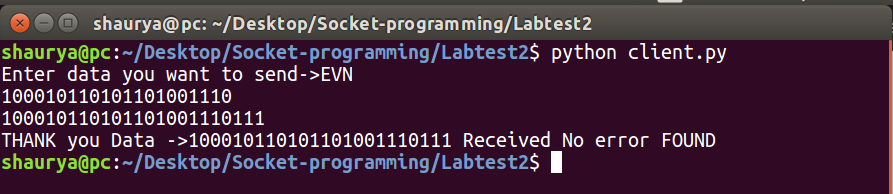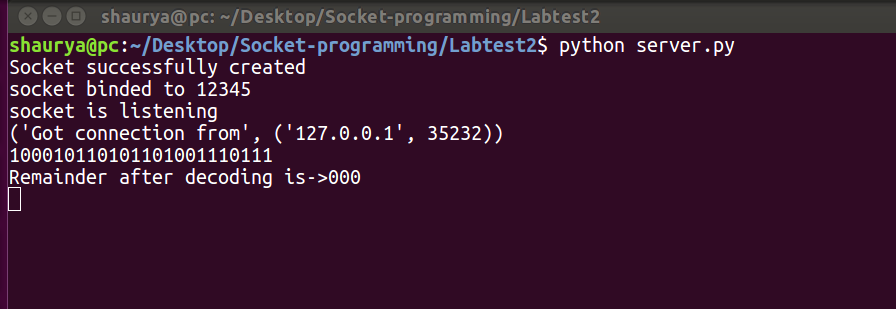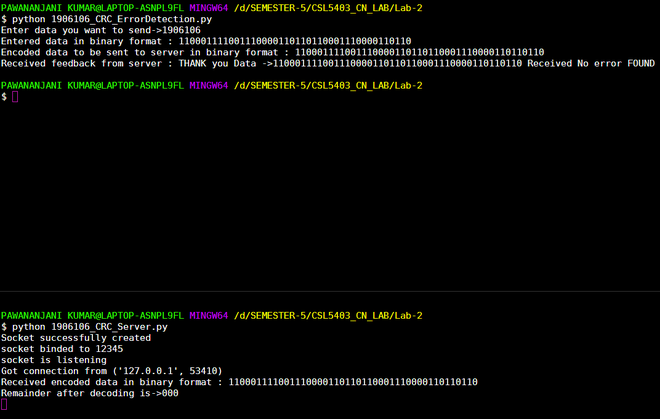GFG App
Open AppBrowser
Continue

Prerequisites: Know about Cyclic redundancy, Socket Programming

What is CRC?
CRC or Cyclic Redundancy Check is a method of detecting accidental changes/errors in the communication channel.
CRC uses Generator Polynomial which is available on both sender and receiver sides. An example generator polynomial is of the form of x^3 + 1. This generator polynomial represents key 1001. Another example is x^2 + x. that represents key 110.

Example:
Let data send is “EVN”
We convert a string to binary string data.

## Python

 `input_string ``=` `"EVN"`   `# CONVERT string data to binary string data` `data ``=` `('``'.join(format(ord(x), '``b') ``for` `x ``in` `input_string))` `print` `(data)`

Output

`100010110101101001110`

CRC KEY: 1001
Code: CRC key length -1 -> 000 appended at end of data.

```New data: 100010110101101001110000
Key:1001```

Now we apply CRC in socket programming python at both sender and receiver sides.

Sender Side

1. The task is to send string data to the server/receiver side.
2. The sender sends a string let us say “EVN”.
3. First, this string is converted to binary string “100010110101101001110” key is known to both the side sender and receiver here key used is 1001.
4. This data is encoded using the CRC code using the key on the client/sender side.
5. This encoded data is sent to the receiver.
6. Receiver later decodes the encoded data string to verify whether there was any error or not.

## Python3

 `# Import socket module` `import` `socket            `   `def` `xor(a, b):`   `    ``# initialize result` `    ``result ``=` `[]`   `    ``# Traverse all bits, if bits are` `    ``# same, then XOR is 0, else 1` `    ``for` `i ``in` `range``(``1``, ``len``(b)):` `        ``if` `a[i] ``=``=` `b[i]:` `            ``result.append(``'0'``)` `        ``else``:` `            ``result.append(``'1'``)`   `    ``return` `''.join(result)`     `# Performs Modulo-2 division` `def` `mod2div(dividend, divisor):`   `    ``# Number of bits to be XORed at a time.` `    ``pick ``=` `len``(divisor)`   `    ``# Slicing the dividend to appropriate` `    ``# length for particular step` `    ``tmp ``=` `dividend[``0` `: pick]`   `    ``while` `pick < ``len``(dividend):`   `        ``if` `tmp[``0``] ``=``=` `'1'``:`   `            ``# replace the dividend by the result` `            ``# of XOR and pull 1 bit down` `            ``tmp ``=` `xor(divisor, tmp) ``+` `dividend[pick]`   `        ``else``: ``# If leftmost bit is '0'`   `            ``# If the leftmost bit of the dividend (or the` `            ``# part used in each step) is 0, the step cannot` `            ``# use the regular divisor; we need to use an` `            ``# all-0s divisor.` `            ``tmp ``=` `xor(``'0'``*``pick, tmp) ``+` `dividend[pick]`   `        ``# increment pick to move further` `        ``pick ``+``=` `1`   `    ``# For the last n bits, we have to carry it out` `    ``# normally as increased value of pick will cause` `    ``# Index Out of Bounds.` `    ``if` `tmp[``0``] ``=``=` `'1'``:` `        ``tmp ``=` `xor(divisor, tmp)` `    ``else``:` `        ``tmp ``=` `xor(``'0'``*``pick, tmp)`   `    ``checkword ``=` `tmp` `    ``return` `checkword`   `# Function used at the sender side to encode` `# data by appending remainder of modular division` `# at the end of data.` `def` `encodeData(data, key):`   `    ``l_key ``=` `len``(key)`   `    ``# Appends n-1 zeroes at end of data` `    ``appended_data ``=` `data ``+` `'0'``*``(l_key``-``1``)` `    ``remainder ``=` `mod2div(appended_data, key)`   `    ``# Append remainder in the original data` `    ``codeword ``=` `data ``+` `remainder` `    ``return` `codeword    ` `    `  `# Create a socket object` `s ``=` `socket.socket()        `   `# Define the port on which you want to connect` `port ``=` `12345`              `# connect to the server on local computer` `s.connect((``'127.0.0.1'``, port))`   `# Send data to server 'Hello world'`   `## s.sendall('Hello World')`   `input_string ``=` `input``(``"Enter data you want to send->"``)` `#s.sendall(input_string)` `data ``=``('``'.join(format(ord(x), '``b') ``for` `x ``in` `input_string))` `print``(``"Entered data in binary format :"``,data)` `key ``=` `"1001"`   `ans ``=` `encodeData(data,key)` `print``(``"Encoded data to be sent to server in binary format :"``,ans)` `s.sendto(ans.encode(),(``'127.0.0.1'``, ``12345``))`     `# receive data from the server` `print``(``"Received feedback from server :"``,s.recv(``1024``).decode())`   `# close the connection` `s.close()`2. Receiver with the help of the key decodes the data and finds out the remainder.
3. If the remainder is zero then it means there is no error in data sent by the sender to the receiver.
4. If the remainder comes out to be non-zero it means there was an error, a Negative Acknowledgement is sent to the sender. The sender then resends the data until the receiver receives the correct data.

## Python3

 `# Import socket module` `import` `socket            `   `def` `xor(a, b):`   `    ``# initialize result` `    ``result ``=` `[]`   `    ``# Traverse all bits, if bits are` `    ``# same, then XOR is 0, else 1` `    ``for` `i ``in` `range``(``1``, ``len``(b)):` `        ``if` `a[i] ``=``=` `b[i]:` `            ``result.append(``'0'``)` `        ``else``:` `            ``result.append(``'1'``)`   `    ``return` `''.join(result)`     `# Performs Modulo-2 division` `def` `mod2div(dividend, divisor):`   `    ``# Number of bits to be XORed at a time.` `    ``pick ``=` `len``(divisor)`   `    ``# Slicing the dividend to appropriate` `    ``# length for particular step` `    ``tmp ``=` `dividend[``0` `: pick]`   `    ``while` `pick < ``len``(dividend):`   `        ``if` `tmp[``0``] ``=``=` `'1'``:`   `            ``# replace the dividend by the result` `            ``# of XOR and pull 1 bit down` `            ``tmp ``=` `xor(divisor, tmp) ``+` `dividend[pick]`   `        ``else``: ``# If leftmost bit is '0'`   `            ``# If the leftmost bit of the dividend (or the` `            ``# part used in each step) is 0, the step cannot` `            ``# use the regular divisor; we need to use an` `            ``# all-0s divisor.` `            ``tmp ``=` `xor(``'0'``*``pick, tmp) ``+` `dividend[pick]`   `        ``# increment pick to move further` `        ``pick ``+``=` `1`   `    ``# For the last n bits, we have to carry it out` `    ``# normally as increased value of pick will cause` `    ``# Index Out of Bounds.` `    ``if` `tmp[``0``] ``=``=` `'1'``:` `        ``tmp ``=` `xor(divisor, tmp)` `    ``else``:` `        ``tmp ``=` `xor(``'0'``*``pick, tmp)`   `    ``checkword ``=` `tmp` `    ``return` `checkword`   `# Function used at the sender side to encode` `# data by appending remainder of modular division` `# at the end of data.` `def` `encodeData(data, key):`   `    ``l_key ``=` `len``(key)`   `    ``# Appends n-1 zeroes at end of data` `    ``appended_data ``=` `data ``+` `'0'``*``(l_key``-``1``)` `    ``remainder ``=` `mod2div(appended_data, key)`   `    ``# Append remainder in the original data` `    ``codeword ``=` `data ``+` `remainder` `    ``return` `codeword    ` `    `  `# Create a socket object` `s ``=` `socket.socket()        `   `# Define the port on which you want to connect` `port ``=` `12345`              `# connect to the server on local computer` `s.connect((``'127.0.0.1'``, port))`   `# Send data to server 'Hello world'`   `## s.sendall('Hello World')`   `input_string ``=` `input``(``"Enter data you want to send->"``)` `#s.sendall(input_string)` `data ``=``('``'.join(format(ord(x), '``b') ``for` `x ``in` `input_string))` `print``(``"Entered data in binary format :"``,data)` `key ``=` `"1001"`   `ans ``=` `encodeData(data,key)` `print``(``"Encoded data to be sent to server in binary format :"``,ans)` `s.sendto(ans.encode(),(``'127.0.0.1'``, ``12345``))`     `# receive data from the server` `print``(``"Received feedback from server :"``,s.recv(``1024``).decode())`   `# close the connection` `s.close()`NOTE:
How to run the program:
1. You should have a socket programming library.

2. First, run server program then runs client program.

3. Indentation error may occur while you copy-paste the code so be careful while copying.

4. You’ll see the following output in your split terminal.My Personal Notes arrow_drop_up